Test: Continuity And Differentiability - 1

# Test: Continuity And Differentiability - 1

Test Description

## 25 Questions MCQ Test Mathematics (Maths) Class 12 | Test: Continuity And Differentiability - 1

Test: Continuity And Differentiability - 1 for Commerce 2022 is part of Mathematics (Maths) Class 12 preparation. The Test: Continuity And Differentiability - 1 questions and answers have been prepared according to the Commerce exam syllabus.The Test: Continuity And Differentiability - 1 MCQs are made for Commerce 2022 Exam. Find important definitions, questions, notes, meanings, examples, exercises, MCQs and online tests for Test: Continuity And Differentiability - 1 below.
Solutions of Test: Continuity And Differentiability - 1 questions in English are available as part of our Mathematics (Maths) Class 12 for Commerce & Test: Continuity And Differentiability - 1 solutions in Hindi for Mathematics (Maths) Class 12 course. Download more important topics, notes, lectures and mock test series for Commerce Exam by signing up for free. Attempt Test: Continuity And Differentiability - 1 | 25 questions in 25 minutes | Mock test for Commerce preparation | Free important questions MCQ to study Mathematics (Maths) Class 12 for Commerce Exam | Download free PDF with solutions
 1 Crore+ students have signed up on EduRev. Have you?
Test: Continuity And Differentiability - 1 - Question 1

###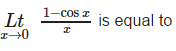Detailed Solution for Test: Continuity And Differentiability - 1 - Question 1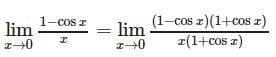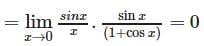Test: Continuity And Differentiability - 1 - Question 2

###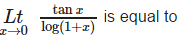Detailed Solution for Test: Continuity And Differentiability - 1 - Question 2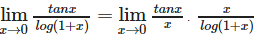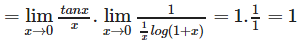Test: Continuity And Differentiability - 1 - Question 3

### Let f(x) = x – [x], then f ‘ (x) = 1 for

Detailed Solution for Test: Continuity And Differentiability - 1 - Question 3

f(x) = x -[x] is derivable at all x ∈ R – I , and f ‘(x) = 1 for all x ∈ R – I .

Test: Continuity And Differentiability - 1 - Question 4

f (x) = max {x, x3},then the number of points where f (x) is not differentiable, are

Detailed Solution for Test: Continuity And Differentiability - 1 - Question 4

f(x)=m{x,x3}
= x;x<−1 and
= x3;−1≤x≤0
⇒ f(x)=x;0≤x≤1 and
= x3;x≥1
∴ f(x)=1;x<−1
∴ f′(x)=3x2;− 1≤x≤0 and =1
0<x<1
Hence answer is 3

Test: Continuity And Differentiability - 1 - Question 5

If f(x) = tan-1x and g(x) =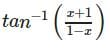, then

Detailed Solution for Test: Continuity And Differentiability - 1 - Question 5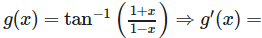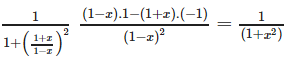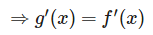Test: Continuity And Differentiability - 1 - Question 6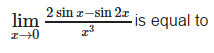Detailed Solution for Test: Continuity And Differentiability - 1 - Question 6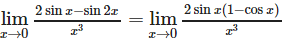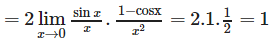Test: Continuity And Differentiability - 1 - Question 7

The function f (x) = 1 + | sin x l is

Detailed Solution for Test: Continuity And Differentiability - 1 - Question 7

f(x) = 1+|sinx| is not derivable at those x for which sinx = 0, however, 1+|sinx| is continuous everywhere (being the sum of two continuous functions)

Test: Continuity And Differentiability - 1 - Question 8

Let f (x + y) = f(x) + f(y) ∀ x, y ∈ R. Suppose that f (6) = 5 and f ‘ (0) = 1, then f ‘ (6) is equal to

Detailed Solution for Test: Continuity And Differentiability - 1 - Question 8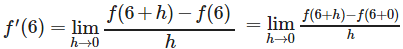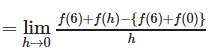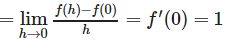Test: Continuity And Differentiability - 1 - Question 9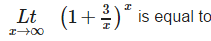Detailed Solution for Test: Continuity And Differentiability - 1 - Question 9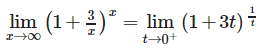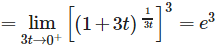Test: Continuity And Differentiability - 1 - Question 10

Derivative of log | x | w.r.t. | x | is

Detailed Solution for Test: Continuity And Differentiability - 1 - Question 10

d/dx(log|x|)
= 1/|x|

Test: Continuity And Differentiability - 1 - Question 11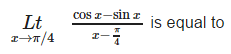Detailed Solution for Test: Continuity And Differentiability - 1 - Question 11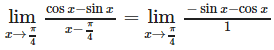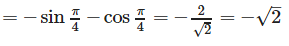Test: Continuity And Differentiability - 1 - Question 12

The function, f (x) = (x – a) sin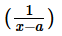for x ≠ a and f (a) = 0 is

Detailed Solution for Test: Continuity And Differentiability - 1 - Question 12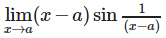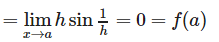Test: Continuity And Differentiability - 1 - Question 13

If x sin (a + y) = sin y, then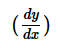is equal to

Detailed Solution for Test: Continuity And Differentiability - 1 - Question 13

x sin(a+y) = sin y
⇒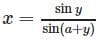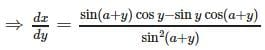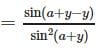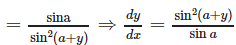Test: Continuity And Differentiability - 1 - Question 14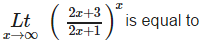Detailed Solution for Test: Continuity And Differentiability - 1 - Question 14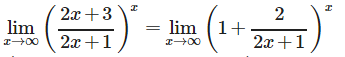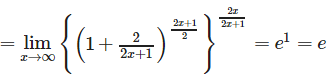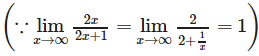Test: Continuity And Differentiability - 1 - Question 15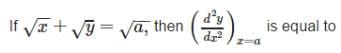Detailed Solution for Test: Continuity And Differentiability - 1 - Question 15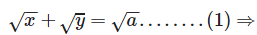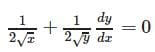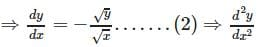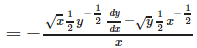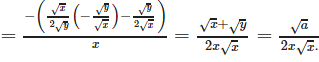Test: Continuity And Differentiability - 1 - Question 16

If [x] stands for the integral part of x, then

Detailed Solution for Test: Continuity And Differentiability - 1 - Question 16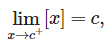If c is an integer , then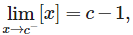Does not exist.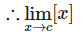Test: Continuity And Differentiability - 1 - Question 17

Let f (x) = [x], then f (x) is

Detailed Solution for Test: Continuity And Differentiability - 1 - Question 17

f(x) = [x] is derivable at all x except at integral points i.e. on R – I .

Test: Continuity And Differentiability - 1 - Question 18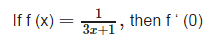Detailed Solution for Test: Continuity And Differentiability - 1 - Question 18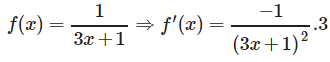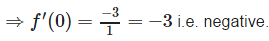Test: Continuity And Differentiability - 1 - Question 19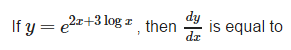Detailed Solution for Test: Continuity And Differentiability - 1 - Question 19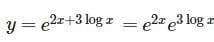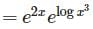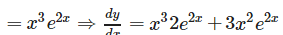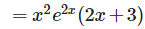Test: Continuity And Differentiability - 1 - Question 20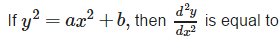Detailed Solution for Test: Continuity And Differentiability - 1 - Question 20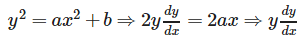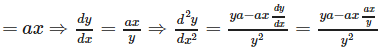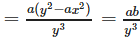Test: Continuity And Differentiability - 1 - Question 21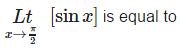Detailed Solution for Test: Continuity And Differentiability - 1 - Question 21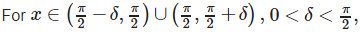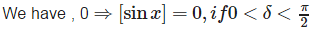Test: Continuity And Differentiability - 1 - Question 22

The function f (x) = [x] is

Detailed Solution for Test: Continuity And Differentiability - 1 - Question 22

The function f (x) = [x] isdiscontinuous only for all integral values of x.

Test: Continuity And Differentiability - 1 - Question 23

Let f be a function satisfying f(x + y) = f(x) + f(y) for all x, y ∈ R, then f ‘ (x) =

Detailed Solution for Test: Continuity And Differentiability - 1 - Question 23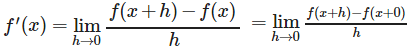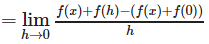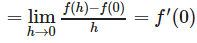Test: Continuity And Differentiability - 1 - Question 24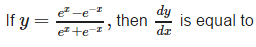Detailed Solution for Test: Continuity And Differentiability - 1 - Question 24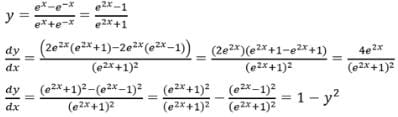Test: Continuity And Differentiability - 1 - Question 25

If x = at2, y = 2at, then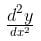is equal to

Detailed Solution for Test: Continuity And Differentiability - 1 - Question 25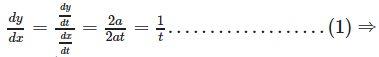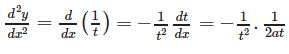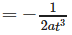## Mathematics (Maths) Class 12

209 videos|209 docs|139 tests
 Use Code STAYHOME200 and get INR 200 additional OFF Use Coupon Code
Information about Test: Continuity And Differentiability - 1 Page
In this test you can find the Exam questions for Test: Continuity And Differentiability - 1 solved & explained in the simplest way possible. Besides giving Questions and answers for Test: Continuity And Differentiability - 1, EduRev gives you an ample number of Online tests for practice

## Mathematics (Maths) Class 12

209 videos|209 docs|139 tests